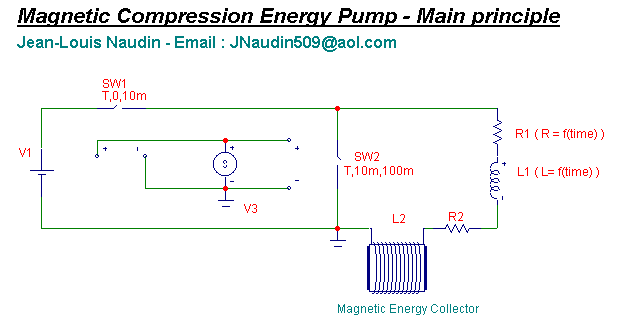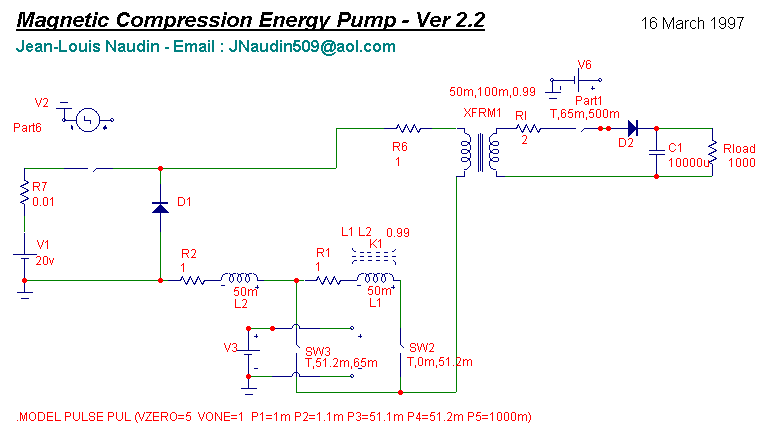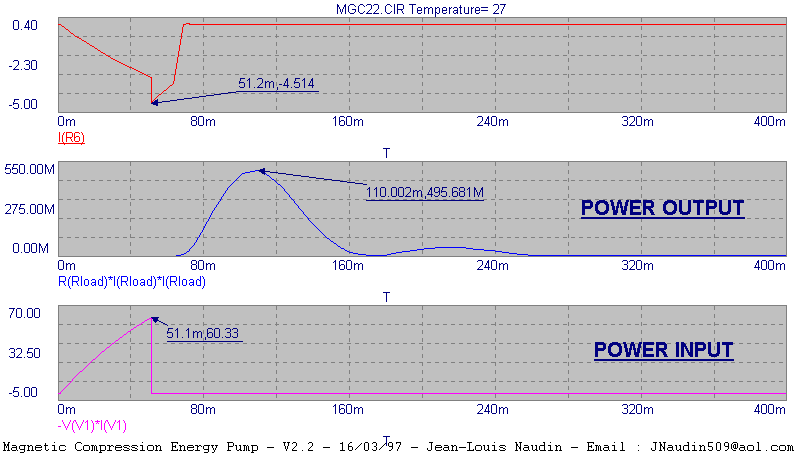The Magnetic Compression Energy Pump ( MCEP project )

This is a thinking about a "Magnetic Compression Energy Pump" device, I look forward to receive your suggestions and comments. Its run on simulation today....and a device soon....

Take a circuit with an inductance L1 (resistance R1) and another inductance L2 (resistance R2). At the beginning L1 and L2 is load with a source and the circuit L1,R1,L2,R2 is closed (disconnect from the source) to start the process.

If we decrease the inductance L1 and its resistance R1 of a circuit rapidly enough to approximately conserve magnetic flux (phi=L1*I1) , we begin a "magnetic compression process". For trying to maintain the flux constant during the magnetic compression process, you must decrease L1 and increase R1, the inductance L2 (R2) is "the collector" and have its characteristics constants.

The circuit must be tuned like this :

- the Tau=L1/R1 must be in function of the time, Tau( at end of process) approximatively equal to 2*Tau.

- at the start of compression :

when we close the circuit we have W(start)=0.5*(L1+L2)*I1^2

(Io is the current when we close the circuit )

- at the end of Magnetic Compression process :

we have L1(end)<<L1(start), R1(end)<<R1(start), Tau(end)=2*Tau(start)

W(end)=0.5*(L1+L2)*I2^2 with I2>>I1 ====>> W(end)>>>W(start)

The "Magnetic Compression Process" run properly IF the decrease of L1 occurs rapidly enough, the damping effect due to the resistance of the circuit is too small to change the magnetic flux appreciably. Since magnetic flux is roughly conserved, the current I will increase as L1 decrease. If the circuit is correctly tuned with the parameters above, the electrical energy produced in the load is higher than the initial electromagnetic energy.You have bellow a simple experimental test circuit :